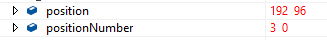# [SOLVED]Isometric 2D game - issue with calculating visible blocks

Hello,
new guy here,
I need help with my code, i’m helpless right now now.

i have my 2D isometric map working but, when i want to implemet mouse selection on each tile it calculates wrongwith a help from : https://gamedevelopment.tutsplus.com/tutorials/creating-isometric-worlds-a-primer-for-game-developers--gamedev-6511

mouse calc Code:

``````public void handleMouse(GameMouse mouse, Camera camera)
{
mousePosition = mouse.state.Position;

Point tile = mousePosition;
tile.X += camera.xOffset;
tile.Y += camera.yOffset;
pointingAt = getTileCoordinates(tile);
int temp = 0;
if (pointingAt.X < 0)
{
temp = pointingAt.X;
pointingAt.X = pointingAt.Y;
pointingAt.Y = -temp;
}
if (pointingAt.Y < 0)
{
temp = pointingAt.Y;
pointingAt.Y = pointingAt.X;
pointingAt.X = -temp;
}

}

public Point getTileCoordinates(Point pt){
Point tempPt = new Point(0, 0);
tempPt.X = (int)Math.Floor((decimal)pt.X / (decimal)(tileHeight / 2+32));
tempPt.Y = (int)Math.Floor((decimal)pt.Y / (decimal)(tileHeight / 2+32));
return(tempPt);
}
``````

and than i just draw basic tile on top of tile that exists:

``````Tile selectTile = tiles.Find(selectedTile => selectedTile.positionNumber.X == (float)pointingAt.X && selectedTile.positionNumber.Y == (float)pointingAt.Y);
if(selectTile != null) {
int x1 = (int)selectTile.positionNumber.X * tileWidth / 2;
int y1 = (int)selectTile.positionNumber.Y * tileHeight / 2;

int ix1 = x1 - y1;
int iy1 = (x1 + y1) / 2;
Point isoCoords1 = new Point(ix1, iy1);
isoCoords1.X -= camera.xOffset;
isoCoords1.Y -= camera.yOffset;
isoCoords1.Y += 48;

Texture2D renderTexture1 = textureList.Find(texture => texture.type.Equals("basic") && texture.id == 999).getTexture2D();
selectTile.drawTile(isoCoords1, renderTexture1, tileWidth, tileHeight/2);
}
``````

when i run the game i’m getting this:

anyone have an idea how to aproach this ?
i will upload full code to GitHub
in zip on github here:

drawing tile on X,Y solved

Well i was changing my rendering of the tiles, i switched to Tile[][] from list.

now i have issue with my calculations:

``````            int si = (-camera.xOffset - tileWidth) / 64;
int ei = (-camera.xOffset + camera.width + tileWidth) / 64;
int sj = (-camera.yOffset - tileHeight) / 64;
int ej = (-camera.yOffset + camera.height + tileHeight) / 64;

Point start = twoDToIso(new Point(si,sj));
Point end = twoDToIso(new Point(ei, ej));

for (int i = start.X; i < end.X; i++)
{
for (int j = start.Y; j < end.Y; j++)
{ //Draw tiles
``````

`

i am lost, and cant sleep becouse of thisThank You.

The first question I would ask is, are you keeping track of the starting coordinates for each tile you have drawn to your map screen?

1 Like

Hi thanks for reply,
yes each tile has its own position (pixel position) and positionNumber(tile number) as seen on picture:Edit: Ha! If i know this i can simlply find the tile where my mouse is… need to to try it in the morning i will post my resul**

## SOLUTION for picking tile on [x][y] in visibleTiles list

**

could not wait for morning so changed my code to :

``````  Tile selectTile = visibleTiles.FindLast(selectedTile =>
(mousePosition.X > selectedTile.position.X && mousePosition.X < selectedTile.position.X + tileWidth / camera.zoom) &&
(mousePosition.Y > selectedTile.position.Y + tileHeight /camera.zoom / 2 && mousePosition.Y < selectedTile.position.Y + tileHeight / camera.zoom));
``````

works like a charm :))

Thank younow i need to optimalize my tiles so i can create more than 490k tiles on 60FPS

Happy I was able to provide you with just enough information to help you resolve your issue…Well i was changing my rendering of the tiles, i switched to Tile[][] from list.

now i have issue with my calculations:

``````            int si = (-camera.xOffset - tileWidth) / 64;
int ei = (-camera.xOffset + camera.width + tileWidth) / 64;
int sj = (-camera.yOffset - tileHeight) / 64;
int ej = (-camera.yOffset + camera.height + tileHeight) / 64;

Point start = twoDToIso(new Point(si,sj));
Point end = twoDToIso(new Point(ei, ej));

for (int i = start.X; i < end.X; i++)
{
for (int j = start.Y; j < end.Y; j++)
{ //Draw tiles
``````

`
i am lost, and cant sleep becouse of this3:30AM niceI am not sure what you are asking here since you said you found the resolution to the issue that started this thread…Sorry i did not explained it right, it is different issue, but i find creating new post unnecessary so here:
i have code that is efficient for 500500 tile map (List solution) with stable FPS but i needed to change it into Tile[x][y], now if i can calculate right way to get tiles that are visible i can get easy map of 50005000 it will be just memory issue but that can be soved by loading chunks ahead of camera movement.

I need to get startx:, startY and endX, endY, if I know the screen width and height, and the camera offset so the result is like this:

(not effective for big maps)

This is my current code:

``````            start = worldToIso(new Point(-camera.xOffset - camera.width /2, camera.yOffset));

if (start.X < 0) start.X = 0;
if (start.Y < 0) start.Y = 0;

for (int i = start.X; i <= start.X + 25; i++)
{
for (int j = start.Y; j <= start.Y +25; j++)
{ // drawTile[i][j];`
``````

This does not work; it will create something like this:

it is OK when i start my map and the origin is  but when i move then it breaks, and my head cant seem to get it.
when i move to the left:

i have uploaded the whole code into gitHub if someone is intrested:

i will keep it up to date as i get some progress1 Like

Hello,
So finally i’m done, had to change my draw approach it was dificult, 3 days of no sleep…
Changed it to ZigZag aproach and it is going very very well, it is harder to select tiles but that just need some time i will figure it outHere screen :

and function video : https://youtu.be/zg5kuiVOm08

i will upload this to git hub if someone is lost like i wasEdit:
Finished picking and with better console !and added map editing in fullscreen !

Video :

2 Likes